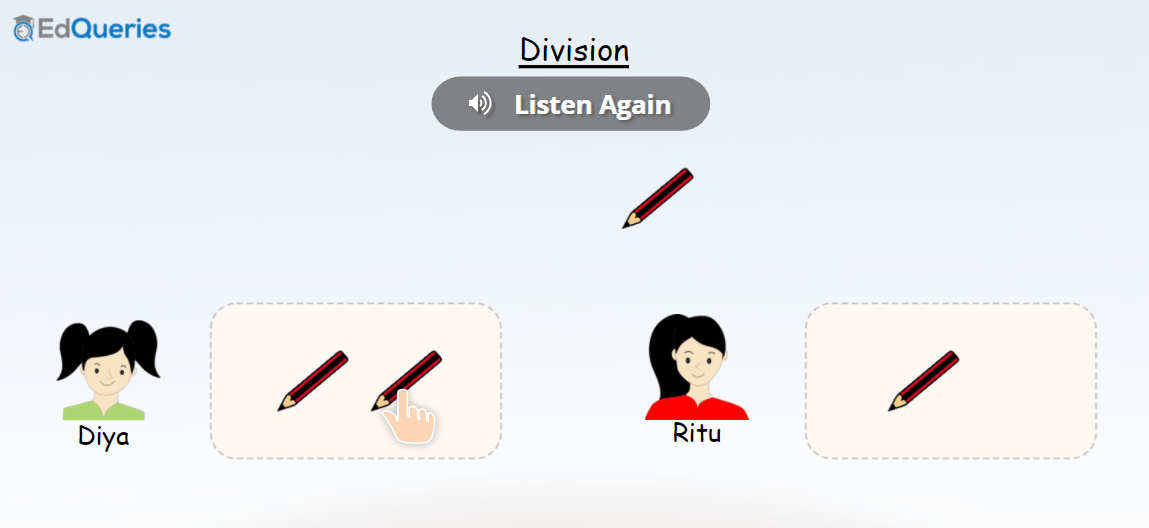# Maths## Learn counting up to 20

In this course, students learn counting. Learning to count is not only about knowing the sequence of numbers, but also knowing the concept of counting. So, in this course, students learn to count by playing various educational games and interactive activities. The course has been broken down into the following sections: To teach the concept …## Learn Counting up to 50

In this course, students learn counting. Learning to count is not only about knowing the sequence of numbers, but also knowing the concept of counting. So, in this course, students learn to count by playing various educational games and interactive activities. The course has been broken down into the following sections: To teach the concept …## Learn Counting up to 10

In this course, students learn counting. Learning to count is not only about knowing the sequence of numbers, but also knowing the concept of counting. So, in this course, students learn to count by playing various educational games and interactive activities. The course has been broken down into the following sections: To teach the concept …## Understanding Fractions

This course is about understanding fractions. This course is an easy to understand fractions. It starts with a ‘Listen and Learn’ activity which presents the concept of fractions visually. Then, there are various ‘Understanding Fractions’ worksheets which are basically interactive activities. These interactive worksheets and educational games are divided into two sections: Understanding Fractions Addition …## Division

This is an online Maths course about Division. It has several educational games and interactive worksheets. These make the topic of division fun and easy to learn. The course starts with a simple explanation about division and has picture based games about division. This online Maths course about division covers division without a remainder as …